## mlcourse.ai – Open Machine Learning Course¶

Author: Denis Mironov (@dmironov)

# Forecasting stock returns with ARIMA, and prices with Ridge and Lasso ¶

## 1. Feature and data explanation¶

### 1.1 Description of the task¶

In the project, we are analyzing five assets:

- Barrick Gold Corporation (ABX)                     Basic Industries
- Walmart Inc. (WMT)                                 Consumer Services
- Caterpillar Inc (CAT)                              Capital Goods
- BP p.l.c. (BP)                                     Energy
- Ford Motor Company (F)                             Capital Goods
- General Electric Company (GE)                      Energy



based on Yahoo Finance (https://finance.yahoo.com) data. In order to reproduce the results of the project, the data for the assets should be either collected manually from Yahoo Finance for the daily time-period from the 10th of December 2013 to 7th of December 2018 or be downloaded here https://github.com/dmironov1993/Data

In this project, our goal is to predict stock returns with Autoregressive Integrated Moving Average (ARIMA) model and stock prices with Ridge and Lasso. ARIMA model is defined by three parameters $(p,d,q)$, where $p$ - the order of the autoregressive model, $d$ - the degree of differencing and $q$ - the order of the moving-average model. The choice of these parameters is done by brute-forcing (grid search) and choosing the model with the lowest Akaike Information Criterion. If time-series is stationary, we are left with p and q parameters, while $d=0$. Such a model is usually called ARMA, and we use this abbreviation hereinafter. However, since Python does not have several important libraries as those in R, for instance the libraries for ARMA-GARCH simultaneous analysis, stock prices will be predicted as well by using machine learning (ML) algorithms such as Ridge and Lasso. At the appropriate stage of our work, we will generate additional features. ML algorithms will be tuned by using grid search of hyperparameters. The performance of ML algorithms will be tested on a houldout sample.

### 1.2. Libraries and Dataset¶

Here we import libraries and load the data in a CSV form which we are going to analysis and working with.

In :
# Jupiter notebook setup and Importing libraries
# By default, all figures are shown in 'png'. If the latter is changed to 'svg', higher quality is guaranteed
%config InlineBackend.figure_format = 'png'
import warnings
warnings.simplefilter('ignore')

# Data manipulations
import pandas as pd
import numpy as np

# Visualization
import seaborn as sns
from matplotlib import pyplot as plt
%matplotlib inline
import plotly
import plotly.graph_objs as go
from plotly import tools
import plotly.plotly as py
init_notebook_mode(connected=True)

# ARIMA (ARMA) modelling
import statsmodels.api as sm
import statsmodels.tsa.api as smt
import statsmodels.tsa.stattools as ts

# Statistics
import scipy.stats as scs
from scipy.stats import skew
from scipy.stats import kurtosis
from statsmodels.tsa.stattools import kpss

# Ljung-box test (to check whether residuals are white noise)
from statsmodels.stats.diagnostic import acorr_ljungbox

# Metrics for ML (ARIMA/ARMA has embedded AIC criterion)
from sklearn.metrics import mean_absolute_error

# Hyperparameter tuning and validation
from sklearn.model_selection import GridSearchCV, TimeSeriesSplit
from sklearn.preprocessing import StandardScaler

# Machine learning algorithms
#from sklearn.linear_model import LassoCV, RidgeCV
from sklearn.linear_model import Ridge, Lasso

In :
# Symbols of assets
asset_names = ['ABX','WMT','CAT','BP','F','GE']

In :
# function for loading csv

"""

Input: list

Output: tuple

"""

ListofAssets_df = []
for asset in symbols:
.sort_values(by='Date', ascending=False))

return tuple(ListofAssets_df)

In :
# number of dataframes within read_csv

6

In :
# number of rows and columns within each df
print (df.shape)

(1258, 7)
(1258, 7)
(1258, 7)
(1258, 7)
(1258, 7)
(1258, 7)

In :
# view of one of dataframes

Out:
Date Open High Low Close ABX_Adj_close Volume
1257 2018-12-07 13.61 13.80 13.49 13.68 13.68 20303800
1256 2018-12-06 13.18 13.48 13.11 13.37 13.37 21323800
In :
# information about structure of data

<class 'pandas.core.frame.DataFrame'>
Int64Index: 1258 entries, 1257 to 0
Data columns (total 7 columns):
Date             1258 non-null object
Open             1258 non-null float64
High             1258 non-null float64
Low              1258 non-null float64
Close            1258 non-null float64
Volume           1258 non-null int64
dtypes: float64(5), int64(1), object(1)
memory usage: 78.6+ KB


We see that there are no missing values in the datasets of our interest.

In the obtained historical prices, we have the following information for each of the asset:

• Date: Date
• Open: Open price within a date
• High: The highest price within a date
• Low: The lowest price within a date
• Close: Close price within a date
• NAME_Adj_close: Adjusted close price in the end of a date

In addition to this information, we are interested in daily returns of the assets. Here the latter will be calculated by using

### $r^{(i)}_{t} := ln\left(\frac{P^{(i)}_{t}}{P^{(i)}_{t-1}} \right) = ln\left( P^{(i)}_{t}\right) - ln\left( P^{(i)}_{t-1} \right),$ ¶

where $ln$ stands for natural logarithm, $P^{(i)}_{t}$ is the adjusted close price of the asset ${i}$ at the time moment ${t}$, while $P^{(i)}_{t-1}$ is at the previous moment of time: ${t-1}$.

In :
# function for adding log-returns column to dataframes

"""
Calculating returns of the assets

Input:
- assets_df: is a tuple of dataframes
- symbols: list with symbols of traded stocks in the same order as those in assets_df
Output:
- tuple of dataframes with returns, dataframe's index is Date now,
dataframe is also sorted by index in ascending order.

"""

ListofAssets_df = []
num_asset = 0

if len(assets_df) == len(symbols):

for df in assets_df:
log_return_array = log_array - np.append(log_array[1:], np.nan)
log_return_df = pd.DataFrame(log_return_array,
columns=['%s_returns' % symbols[num_asset]])
df = df.reset_index().drop(columns=['index'])
df = pd.concat((df, log_return_df), axis=1)
df['Date'] = df['Date'].apply(pd.to_datetime)
df = df.set_index('Date')
df = df.sort_index(ascending=True)
df.dropna(axis=0, inplace=True)
ListofAssets_df.append(df)
num_asset += 1

return tuple(ListofAssets_df)

else:
print ('Number of DataFrames and Number of assets considered should be equal')

In :
asset_dfs = add_log_returns(read_csv(asset_names), asset_names)

In :
# we have added returns, year, month and day columns
for df in asset_dfs:
print (df.shape)

(1257, 7)
(1257, 7)
(1257, 7)
(1257, 7)
(1257, 7)
(1257, 7)

In :
asset_dfs.head(3)

Out:
Open High Low Close ABX_Adj_close Volume ABX_returns
Date
2013-12-11 16.850000 16.879999 16.32 16.379999 15.428679 15990000 -0.029476
2013-12-12 15.950000 16.510000 15.94 16.459999 15.504032 15321000 0.004872
2013-12-13 16.639999 16.980000 16.51 16.740000 15.767772 15426700 0.016868

Now each of the dataframes, in addition to already presented six columns such as Open, High, Low, Close, NAME_Adj_close and Volume, has one additional column related to returns. Note that Date is index now rather than a column. Dataframes have been sorted by Date in ascending order. The latter is needed further for correct time series cross validation. Number of rows has been reduced to 1257 (1258 originally) since our data does not allow to calculate returns before 11th of December 2013.

For convenience, we present two functions. One to create a dataframe consisting of only adjusted close prices of our assets, while the other one is to create a dataframe consisting of only asset returns.

In :
def asset_adj_close(list_of_df, symbols):
"""

Input:
- list_of_df: list of dataframes. Each of the latter should have
a column corresponding to adjusted close price
- symbols: list of asset symbols taken from a stock market
Output:
- pandas dataframe consisting of only adjusted close prices of considered assets

"""

number = 0
for asset in asset_names:
number += 1


In :
adj_close = asset_adj_close(asset_dfs, asset_names)

Out:
Date
2013-12-11 15.428679 69.464432 71.602913 34.299854 13.081720 22.148977
2013-12-12 15.504032 68.946243 71.846390 33.910583 13.065777 22.115643
In :
def asset_returns(list_df, symbols):
"""

Input:
- list_df: list of dataframes each of which contains asset returns column
- symbols: list of asset symbols taken from a stock market
Output:
- a dataframe consisting of only asset returns

"""

asset_returns = []
k = 0
for asset in symbols:
asset_returns.append(list_df[k]['%s_returns' % asset])
k += 1

return pd.concat(asset_returns, axis=1)

In :
returns = asset_returns(asset_dfs, asset_names)

In :
returns.head(2)

Out:
ABX_returns WMT_returns CAT_returns BP_returns F_returns GE_returns
Date
2013-12-11 -0.029476 0.000127 -0.013279 0.002787 -0.007286 -0.020850
2013-12-12 0.004872 -0.007488 0.003395 -0.011414 -0.001219 -0.001506

Next we perform primary visual data analysis of adjusted close prices and returns.

## 2. Primary data analysis, Primary visual data analysis, Insights and found dependencies¶

The structure of this section as follows

• Correlation and Pairplot
• A normal quantile-quantile plot and Comparison of their Kernel Density Estimation (KDE) to the closest parametric normal distribution
• Skewness, Kurtosis, Max value, Min value, Mean and Variance
• Box plot
• Time Series plot
• Correlation function and Partial correlation function

### 2.1. Correlation and Pairplot¶

Here we provide a correlation table and a scatter plot against each other for both prices and returns of Barrick Gold Corporation (ABX), Walmart Inc. (WMT), Caterpillar Inc (CAT), BP p.l.c. (BP), Ford Motor Company (F) and General Electric Company (GE). Comparison of Pearson and Spearman correlations let us know the affection of large outliers on general picture of the assets movement.

First we present two auxiliary functions

In :
def corr_plot(df, symbols, days=252, title='Returns'):

"""
Input:
- df: a dataframe consisting only of data which correlations will be plotted
- symbols: a list of the companies stocks names
- days: represent the number of days to the past, set it to 0 to get consider the whole range
- title: the plot title in accordance with df
Output:
- illustration of assets correlations

"""

fig, axes = plt.subplots(nrows=1, ncols=2, figsize=(20,8))
sns.heatmap(df[-days:].corr(method='pearson'), annot=True, fmt='.2f', cmap="YlGnBu",
xticklabels=symbols, yticklabels=symbols, ax=axes)
sns.heatmap(df[-days:].corr(method='spearman'), annot=True, fmt='.2f', cmap="YlGnBu",
xticklabels=symbols, yticklabels=symbols, ax=axes)
axes.set_title('Pearson correlation (%s)' % title)
axes.set_title('Spearman correlation (%s)' % title)
plt.show()

In :
def pairplot(df, days=252, width=9, height=9):

"""
Input:
- df: a dataframe consisting only of data which will be plotted
- width and height: a corresponding size of the plot
Output:
- illustration of assets pairplot

"""
g = sns.pairplot(df[-days:]);
g.fig.set_size_inches(width,height)
plt.show()


#### 2.1.1 Asset Returns¶

In :
corr_plot(returns, asset_names, title='Returns')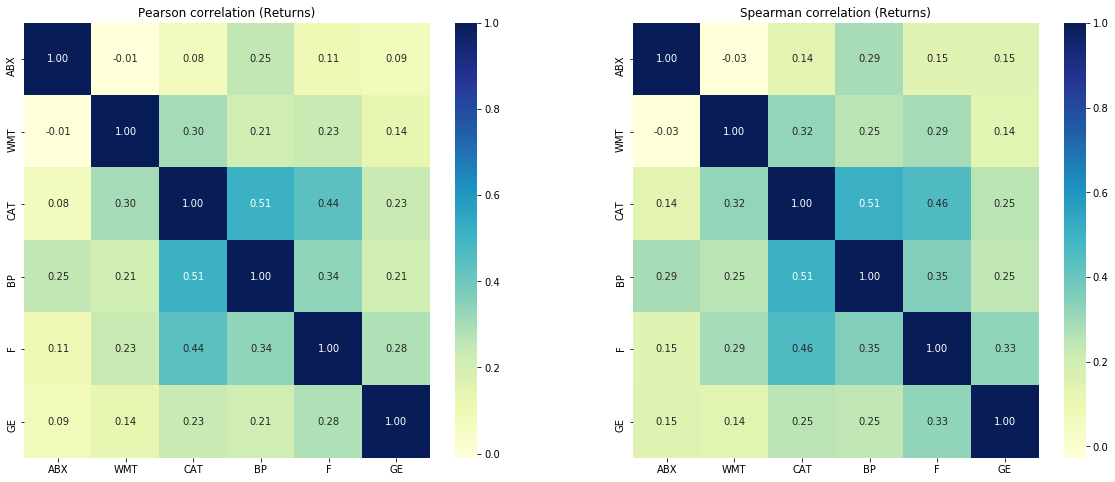From the figure right above one can see that correlation between asset returns does not change much between two types of correlations. The returns tend to comoving within 252 days time interval.

Now let's take a look at the whole picture of pair-correlations.

In :
pairplot(returns, width=9, height=9)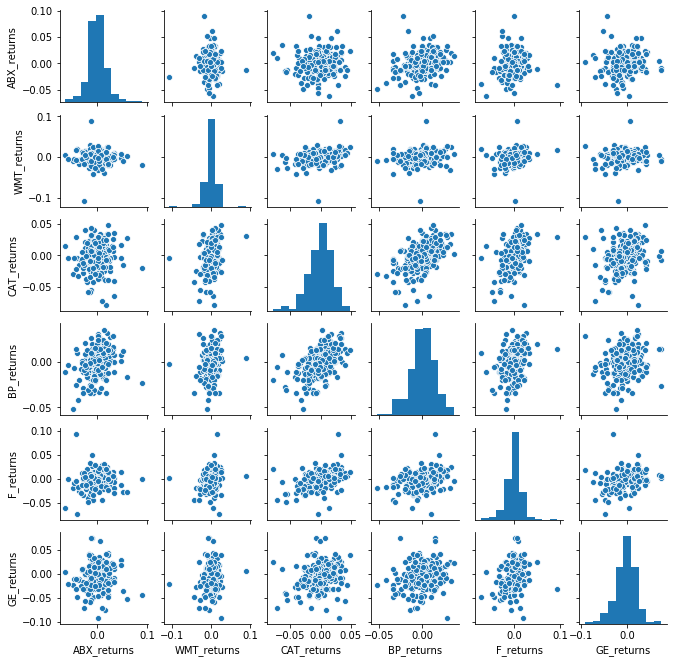#### 2.1.2 Asset Prices¶

In :
corr_plot(adj_close, asset_names, title='Adj close price')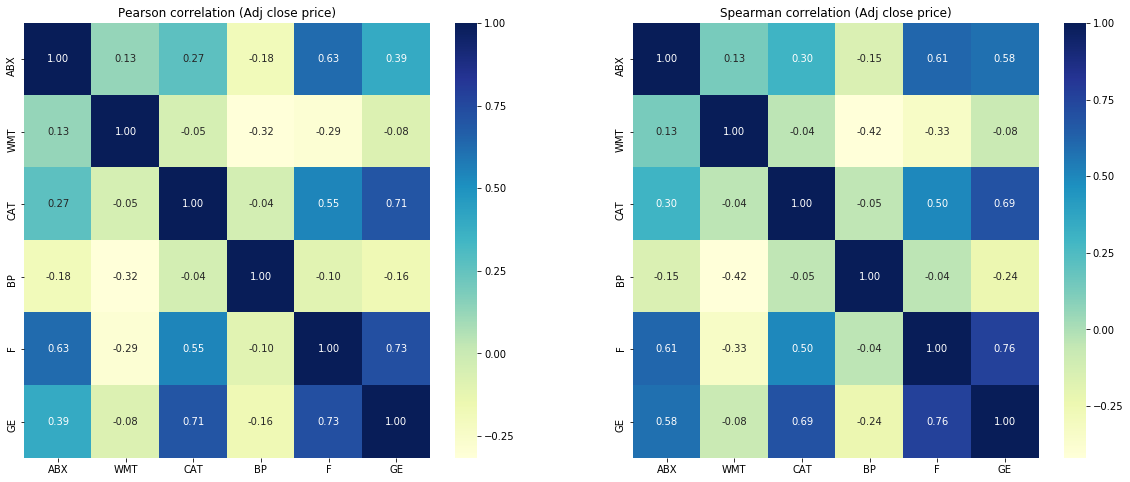In the figure right above, Pearson correlation for asset adjusted close price is demonstrated on the left, while Spearman correlation is on the right. We see that some of the prices tend to comoving while others moving in opposite directions.

In :
pairplot(adj_close, width=9, height=9)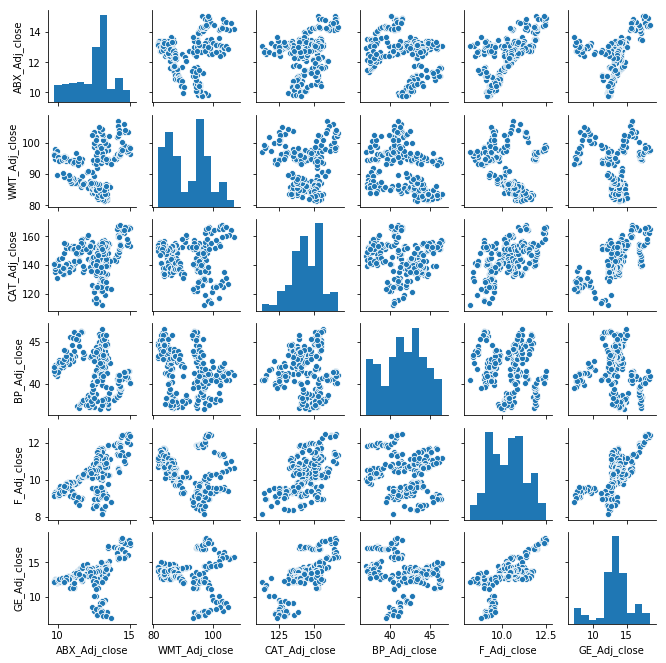The above is the scatter plot for adjusted close prices. This allows us to see general tendency of asset pairs.

According to the correlation analysis, the assets considered here tend to comoving. However, we should also remember what time-interval is considered. Here we take into account 252 days interval corresponding to about 1 trading year. If some other time interval is of interest, then correlation coefficients for all of the assets change appropriately. It is not possible to know in advance whether the correlation between the assets will be higher, remain the same or lower with changing a time-period. But such an analysis demonstrates an estimation of their possible correlation values.

### 2.2. A normal quantile-quantile plot and Comparison of their Kernel Density Estimation (KDE) to the closest parametric normal distribution¶

In this section, we discuss return and adj close price distributions of United Continental Holdings Inc. (UAL), BP p.l.c. (BP), American Water Works Company Inc. (AWK), Ford Motor Company (F), General Electric Company (GE) and Walmart Inc. (WMT).

As previously, we introduce several auxiliary functions first.

In :
def norm_function(x, mu, sigma):
return (1/(sigma*np.sqrt(2*np.pi))) * np.exp(-(x-mu)**2 / (2*sigma**2))

In :
def qqplot_returns(df, symbols, option='returns', size=(10,5), sharex=False, sharey=False, wspace=0.6, hspace=0.4):

"""

Input:
- df:
- symbols:
- size:
- sharex:
- sharey:
- wspace:
- hspace:

Output:
- illustration of a normal quantile-quantile plot of returns

"""
nrows = len(symbols)
if nrows != 1:
fig, axes = plt.subplots(nrows=nrows, ncols=2, sharex=sharex, sharey=sharey, figsize=size)
for i in range(nrows):

if option == 'returns':
name = '%s' % symbols[i]
name_return = '%s_returns' % symbols[i]
name = '%s' % symbols[i]

probplot = sm.ProbPlot(df[name_return], dist='norm')
fig = probplot.qqplot(line='q', ax=axes[i,0])
axes[i,0].set_title('Normal Q-Q Plot (%s)' % name)

sns.distplot(df[name_return], kde=True, hist=False, ax=axes[i,1], color='black')
count, mean, std, min_, q1, mean, q3, max_ = df[name_return].describe()
xx = np.linspace(min_, max_, 1000)
axes[i,1].plot(xx, norm_function(xx, mean, std), '--', color='red')
axes[i,1].set_title(name)
axes[i,1].set_xlabel('')
plt.show()

else:
if option == 'returns':
name = '%s' % symbols
name_return = '%s_returns' % symbols
name = '%s' % symbols

nrows = 1
fig, axes = plt.subplots(nrows=nrows, ncols=2, sharex=sharex, sharey=sharey, figsize=size)
name = '%s' % symbols
name_return = '%s_returns' % symbols

probplot = sm.ProbPlot(df[name_return], dist='norm')
fig = probplot.qqplot(line='q', ax=axes)
axes.set_title('Normal Q-Q Plot (%s)' % name)

sns.distplot(df[name_return], kde=True, hist=False, ax=axes, color='black')
count, mean, std, min_, q1, mean, q3, max_ = df[name_return].describe()
xx = np.linspace(min_, max_, 1000)
axes.plot(xx, norm_function(xx, mean, std), '--', color='red')
axes.set_title(name)
axes.set_xlabel('')
plt.show()


#### 2.2.1 Asset Prices¶

In :
qqplot_returns(adj_close, asset_names, option='adj_close',
size=(10,20), sharex=False, sharey=False, wspace=0.6, hspace=0.6)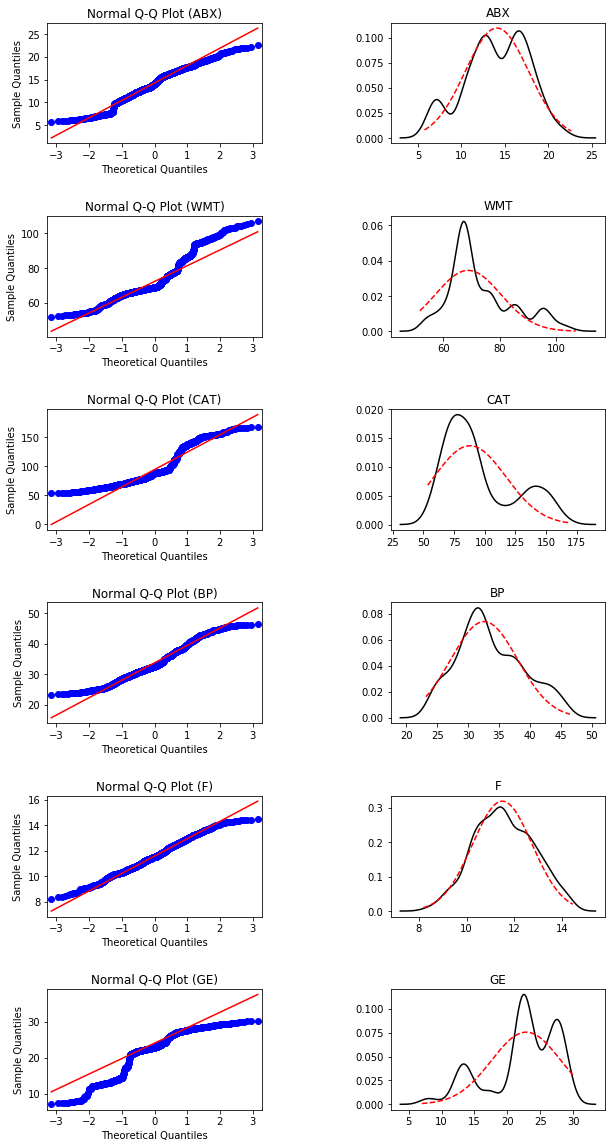On the left-hand side the normal quantile-quantile plots are shown, while on the right-hand side kernel density estimate of returns and their closest normal distribution are illustrated. Red lines correspond to normal distribution.

We see that some of adjusted close prices have bi-modal distributions while some others have even more complex structure which does not appear to be normal even close.

#### 2.2.1 Asset Returns¶

In :
qqplot_returns(returns, asset_names, option='returns',
size=(10,20), sharex=False, sharey=False, wspace=0.6, hspace=0.6)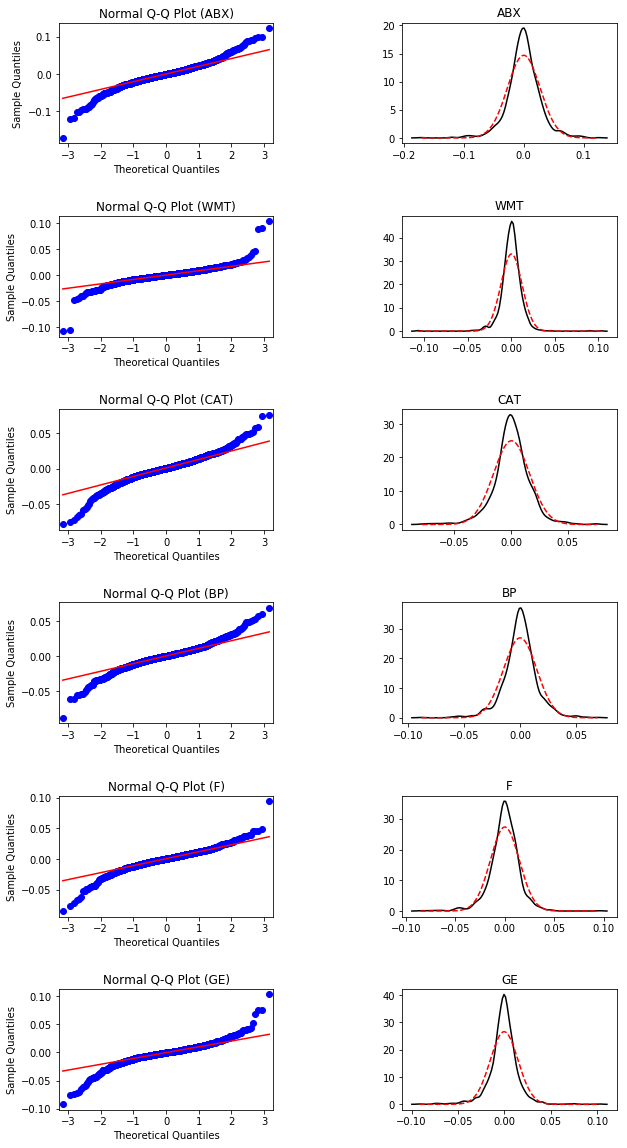Red lines correspond to normal distribution. Note that the distribution is not normal as demonstrated by both kinds of plots demonstrating fatter tails and higher kurtosis. However, their structure is closer to the normal distribution than that of adjusted close price.

On this stage of the work we may colcude that fat tails will become a problem for our ARIMA or ARMA modelling since it may be that we will not encompass all the time-series information due to that. Let's keep this notion in mind and move on.

### 2.3. Skewness, Kurtosis, Max value, Min value, Mean and Variance¶

Now let's gather more statistics about target values. Precisely speaking, their Skewness, Kurtosis, Max returns, Max loss, Mean and Variances.

In :
def stats(df, symbols):
"""

Input:
- symbols: a list of asset symbols

Output:
- a dataframe containing information such as Skewness, Kurtosis, Max value,
Min value, Mean and Variance of the df

"""

stat = pd.DataFrame(index=asset_names,
columns=['Skewness','Kurtosis','Max value',
'Min value','Mean','Variance'])

stat['Skewness'] = skew(df, axis=0)
stat['Kurtosis'] = kurtosis(df, axis=0)
stat['Max value'] = df.agg('max').values
stat['Min value'] = df.agg('min').values
stat['Mean'] = df.agg('mean').values
stat['Variance'] = df.agg('var').values

return stat

In :
stats(adj_close, asset_names)

Out:
Skewness Kurtosis Max value Min value Mean Variance
ABX -0.296287 -0.538592 22.630032 5.750702 14.059425 13.234067
WMT 0.825894 -0.011779 107.010101 51.773445 73.242156 133.737496
CAT 0.834336 -0.561894 167.927460 53.464493 95.752004 855.647013
BP 0.360279 -0.583571 46.459087 23.173573 33.660785 29.222048
F 0.039854 -0.512979 14.447714 8.180000 11.576889 1.569655
GE -0.850812 -0.110201 30.092396 7.010000 22.347276 27.903293
In :
stats(returns, asset_names)

Out:
Skewness Kurtosis Max value Min value Mean Variance
ABX -0.231618 3.397397 0.123010 -0.170784 -0.000119 0.000739
WMT -0.152985 18.770065 0.103444 -0.107399 0.000234 0.000146
CAT -0.291100 3.134340 0.075671 -0.078606 0.000423 0.000255
BP -0.203216 3.168546 0.069155 -0.088332 0.000115 0.000220
F -0.555645 4.667572 0.094421 -0.085174 -0.000319 0.000213
GE -0.331204 6.558640 0.102597 -0.091911 -0.000932 0.000225

### 2.4. Box plot of Returns¶

Box plot will help us to get the precise information about outliers and how they fit in the whole picture.

In :
def asset_box_plot(df, symbols, title=None, width=700, height=400,
jitter=0.2, pointpos=-1.5, boxpoints = 'suspectedoutliers'):
"""

Input:
- df: a dataframe which columns will be plotted,
- symbols: a list of symbols in the order the same as that in dataframe.
- title:
- width:
- height:
- jitter:
- pointpos:

Output:
- Box plot illustrated by plotly library

"""

data=[]
for i in range(len(symbols)):
trace = go.Box(y = df.iloc[:,i],
name = symbols[i],
jitter=jitter,
pointpos=pointpos,
boxpoints = boxpoints)

data.append(trace)

layout = go.Layout(title = title,
autosize=False,
width=width,
height=height)

fig = go.Figure(data=data,layout=layout)

iplot(fig)


### 2.4.1 Asset Price¶

In :
asset_box_plot(adj_close, asset_names, title='Adj close price', boxpoints = 'suspectedoutliers')


### 2.4.2 Asset Returns¶

In :
asset_box_plot(returns, asset_names, title='Returns')